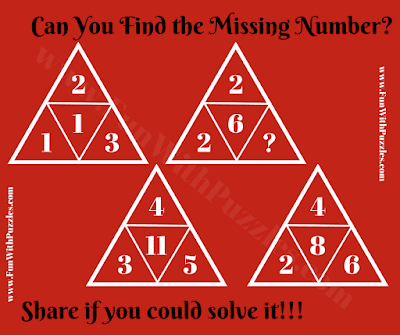## Thursday, August 20, 2020

Here is Missing Number in Triangle Puzzle. In this puzzle, you are given 4 numbers inside four triangles. These numbers inside each of the triangles relate to each other with some Mathematical formula. Your challenge is to find this relationship among these numbers and then find the value of the missing number which will replace the question mark. So can you find this missing number in the triangle?Can you find the missing number in Triangle?

The answer to this "Missing Number in Triangle Puzzle", can be viewed by clicking on the button. Please do give your best try before looking at the answer.

1.2.